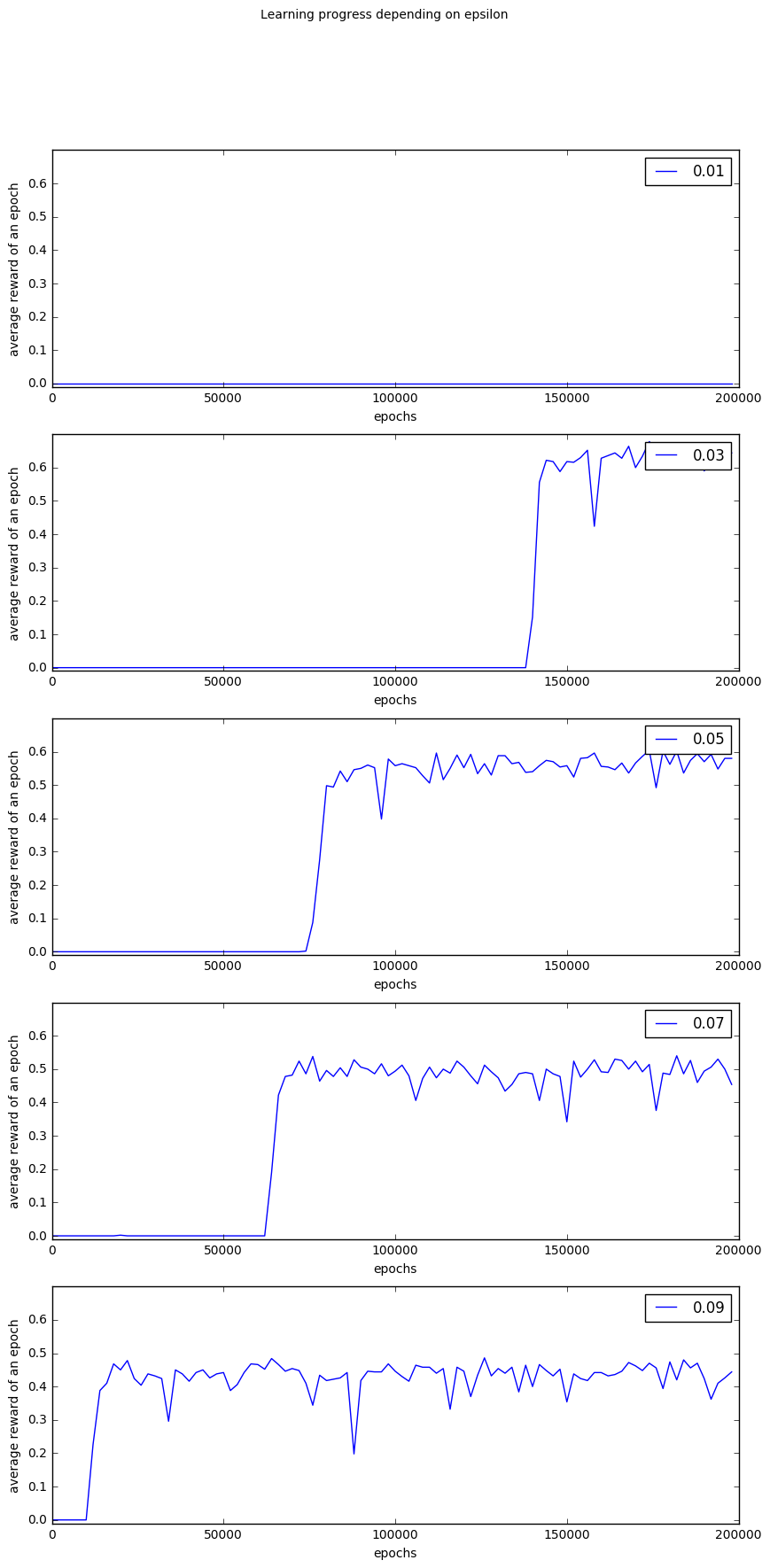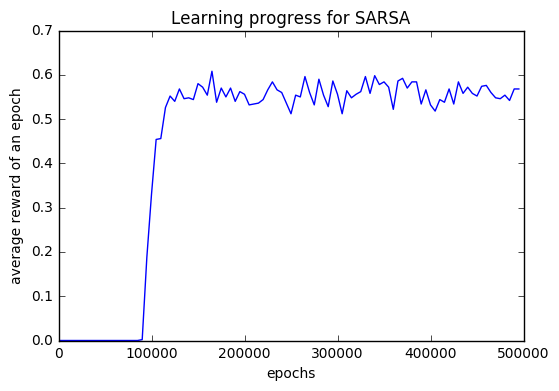temporal_difference_learning slides

# Temporal-Difference (TD) Learning¶

here only TD(0)

### Temporal-Difference Learning¶

• TD methods learn directly from episodes of experiments
• TD is model-free: no knowledge of MDP transitions / rewards
• TD learns from incomplete episodes, by bootstrapping
• TD updates a guess towards a guess

## TD-Predition¶

from [SuBa] 6.1 TD Prediction

General Rule: We move the estimate towards the target:

$$\text{newEstimate} \leftarrow \text{oldEstimate} + \alpha (\text{Target} - \text{oldEstimate} )$$

#### Constant $\alpha$ MC-Update (every visit):¶

We write $s_t$ as an abbreviation for $S_t = s$ and $s'_{t+1}$ for $S_{t+1}=s'$.

$$v(s_t) \leftarrow v(s_t) + \alpha (\mathcal R_{t} - V(s_t))$$

with

• $\mathcal R_t$: actual return following time $t$
• $v(s_t)$: Value function of the state at time $t$

#### TD-Update for $v$:¶

Update value $v(s_t)$ towards estimated return $R_{t+1} + \gamma V(s_{t+1})$:

$$v(s) \leftarrow v(s) + \alpha \left(R_{t+1} + \gamma V(s'_{t+1}) - V(s_t)\right)$$

with

• $R_{t+1}$: reward after
• $v(s_t)$: Value function of the state at time $t$

• $R_{t+1} + \gamma V(s_{t+1})$ is called the TD target

• $\delta_t = R_{t+1} + \gamma V(s_{t+1}) - V(s_t)$ is called the TD error

Bootstrapping: update is based partially on an existing estimate.

TD methods combine the sampling of Monte Carlo with the bootstrapping of DP.

In :
import gym
env = gym.make('FrozenLake-v0')
env.action_space

[2016-11-25 10:59:55,816] Making new env: FrozenLake-v0

Out:
Discrete(4)
In :
env.observation_space

Out:
Discrete(16)
In :
env.step(0)

Out:
(0, 0.0, False, {'prob': 0.3333333333333333})

## Sarsa - On Policy TD Control¶

SARSA: State-Action Reward State-Action

#### TD-Update for $q$:¶

$$q(s_t, a_t) \leftarrow q(s_t, a_t) + \alpha \left(R_{t+1} + \gamma q\left(s'_{t+1}, \pi(s')\right) - q(s_t, a_t) \right)$$

with

• $R_{t+1}$
• $q(s_t, a_t)$: Value function of the state at time $t$

Bootstrapping: update is based partially on an existing estimate.

TD methods combine the sampling of Monte Carlo with the bootstrapping of DP.

In :
# SARSA: On-policy TD control algorithm
def sarsa(alpha = 0.02,
gamma = 1.,
epsilon = 0.05,
q = None,
progress = None,
env=env):

if q is None:
q = np.ones((16,4))

for i in range(nb_episodes):
done = False
s = env.reset()
a = action_epsilon_greedy(q, s, epsilon=epsilon)
while not done:
new_s, reward, done, info = env.step(a)
new_a = action_epsilon_greedy(q, new_s, epsilon=epsilon)
q[s, a] = q[s, a] + alpha * (reward + gamma * q[new_s, new_a] - q[s, a])
s = new_s
a = new_a

# only for plotting the performance, not part of the algorithm
if progress is not None and i%STEPS == 0:
progress[i//STEPS] = average_performance(get_action_epsilon_greedy(epsilon), q=q)
return q, progress

In :
plot_performance(param_performance)As you can see the larger $\epsilon$ the faster is the learning. But the larger the $\epsilon$ the lower is the average reward per episode.

A hack could be to use at the end the greedy policy. But keep in mind, that we have not estimated the q-values of the greedy policy. So this can be problematic in more elaborate environments.

In :
plt.plot(STEPS*np.arange(nb_episodes//STEPS), sarsa_performance)
plt.xlabel("epochs")
plt.title("Learning progress for SARSA")
plt.ylabel("average reward of an epoch")

Out:
<matplotlib.text.Text at 0x10b015a90>In :
def greedy_policy(q, s):
return np.argmax(q[s])
print(average_performance(greedy_policy, q=q))

0.838


## Q-Learning - Off Policy Control¶

Read [SuBa] 6.5 Q-Learning: Off-Policy TD Control

Update rule:

$$q(s_t, a_t) \leftarrow q(s_t, a_t) + \alpha \left(R_t + \gamma \cdot \text{arg}\max_{a'} \left(q\left(s'_{t+1}, a'\right)\right) - q(s_t, a_t) \right)$$

Here:

• estimation policy is greedy
• behaviour policy is $\epsilon$-greedy
In :
# Q-Learning: Off-policy TD control algorithm
for i in range(nb_episodes):
done = False
s = env.reset()
while not done:
a = action_epsilon_greedy(q, s) # behaviour policy
new_s, reward, done, info = env.step(a)
a_max = np.argmax(q[new_s]) # estimation policy
q[s, a] = q[s, a] + alpha * (reward + gamma * q[new_s, a_max] - q[s, a])
s = new_s

# for plotting the performance
if i%STEPS == 0:
q_performance[i//STEPS] = average_performance(greedy_policy, q)

In :
average_performance(greedy_policy, q)

Out:
0.828

• return $G_t = R_{t+1}+ \gamma R_{t+2} + \dots + \gamma^{T-1}R_T$ is unbiased estimate of $v_\pi(S_t)$
• true TD target $R_{t+1} + \gamma v_\pi (S_{t+1})$ is unbiased estimate of $v_\pi(S_t)$
• TD target $R_{t+1} + \gamma V_\pi (S_{t+1})$ is biased estimate of $v_\pi(S_t)$
• TD target is much lower variance than the return:
• return depends on many random actions, transitions, rewards
• TD target depends on one random action, transition, reward

• TD can learn before knowing the final outcome
• TD can learn online after every step
• MC must wait until end of episode before return is known
• TD can learn without the final outcome
• TD can learn from incomplete sequences
• MC can only learn from complete sequences
• TD works in continuing (non-termiating) environments
• MC only works for episodic (terminating) environments

• return $G_t = R_{t+1}+ \gamma R_{t+2} + \dots + \gamma^{T-1}R_T$ is unbiased estimate of $v_\pi(S_t)$
• true TD target $R_{t+1} + \gamma v_\pi (S_{t+1})$ is unbiased estimate of $v_\pi(S_t)$
• TD target $R_{t+1} + \gamma V_\pi (S_{t+1})$ is biased estimate of $v_\pi(S_t)$
• TD target is much lower variance than the return:
• return depends on many random actions, transitions, rewards
• TD target depends on one random action, transition, reward

• MC has high variance, zero bias
• good convergence properties
• (even with function approximation)
• not very sensitive to initial value
• very simple to understand and use
• TD has low variance, some bias
• usually more efficent than MC
• TD(0) converges to $V_\pi(s)$
• (but not always with function approximation)
• more sensitive to initial values

#### Certainty Equivalence¶

• MC converges to solution with minimum mean-squared error
• best fit to the observed returns $$\sum_{k=1}^K \sum_{t=1}^{T_k} \left(g_t^k - V(s_t^k)\right)$$
• TD(0) converges to solution of max likelihood Markov model
• solution to the MDP $\left< \mathcal S, \mathcal A, \mathcal{\hat P}, \mathcal{\hat R}, \gamma \right>$ that best fits the data $$\mathcal{\hat P^a_{s,s'}} = \frac{1}{N(s,a)}\sum_{k=1}^{K}\sum_{t=1}^{T_k}\mathbb 1(s_t^k, a_t^k,s_{t+1}^k=s,a,s')$$ $$\mathcal{\hat R^a_{s,s'}} = \frac{1}{N(s,a)}\sum_{k=1}^{K}\sum_{t=1}^{T_k}\mathbb 1(s_t^k, a_t^k=s,a)r_t^k$$

• TD exploits Markov property
• usually more efficient in Markov environments
• MC does not exploid Markov peoperty
• usually more effective in non-Markov environments

#### Bootstapping and Sampling¶

• Bootstrapping: update involves an estimate
• MC does bootstrap
• DP bootstraps
• TD bootstraps
• Sampling:
• MC samples
• DP does not sample
• TD samples